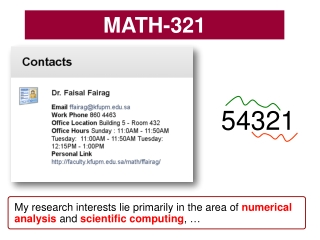DownloadDownload PresentationMATH-321

# MATH-321

Download Presentation## MATH-321

- - - - - - - - - - - - - - - - - - - - - - - - - - - E N D - - - - - - - - - - - - - - - - - - - - - - - - - - -
##### Presentation Transcript

1. MATH-321 54321 My research interests lie primarily in the area of numerical analysis and scientific computing, …

2. Numerical analysis Math 321: Introduction to Numerical Computing Numerical analysis the branch of mathematics that deals with the development and use of numerical methods for solving problems. “Solve” problems with no analytic solution Numerical analysis naturally finds applications in all fields of engineering, the physical sciences, life sciences, …..

3. MATH-321 SYLLABUS 2) Polynomial interpolation 4) Numerical integration and differentiation* 1) Solution of non-linear equations 7) Linear Regression 3) Splines 6) Solution of linear algebraic systems 5) IVB & BVP* Worked: Num. Sol. for PDE. Accele linear and nonlinear solver and their error analysis 8) Error Analysis

4. MATH-321 1)Request 2)Messages 3)BBQ 1)Webpage 2)Resources

5. MATH-321 SYLLABUS 2) Polynomial interpolation 4) Numerical integration and differentiation* 1) Solution of non-linear equations 7) Linear Regression 3) Splines 6) Solution of linear algebraic systems 5) IVB & BVP* Computer Usage: Computer software is essential for this course. Mainly we will be using MATLAB as the computational platform 8) Error Analysis

6. Quick Introduction to Matlab

7. Script file in Matlab Example Solve the quadratic equation

8. Script file in Matlab Example Solve the quadratic equation Type name of the file

9. Script file in Matlab Example Solve the quadratic equation